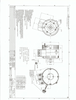# ME1114

## Product Description

Output Power of 10 KW Continuous, 24 KW Peak (at 72 volts)

Designed for long life. No brush maintenance. The motor is 92% efficient at voltages between 24 to 72 VDC. Continuous current of 200 Amps. This is a 3-phase, Y-connected Permanent Magnet Synchronous Motor with an axial air gap and Sine/Cosine Speed Sensor. It has two stators with a rotor in the center.

1) This is a 4 pole motor (8 magnets). 2) The Phase to Phase winding resistance is 0.065 Ohms with 20 turns per phase. 3) The maximum recommended rotor speed is 5000 RPM. 4) Voltages from 0 to 72 VDC input to the control. 5) Torque constant of 0.12 Nm per Amp 6) The Inductance Phase to Phase is 0.05 Milli-Henry. 7) Armature Inertia is 45 Kg Cm Squared. 8) Continuous current of 200 Amps AC. 9) Peak current of 420 Amps AC for 1 minute. 10) Weight of 29 pounds. 11) Peak Stall Torque if 48 ft lb. 12) This is an Open Frame, Fan Cooled motor.

Includes temperature sensor.ME1114 Rev.A Drawing